Courses

# NCERT Exemplar: Pair of Linear Equations in Two Variables Notes | EduRev

## Class 10 : NCERT Exemplar: Pair of Linear Equations in Two Variables Notes | EduRev

The document NCERT Exemplar: Pair of Linear Equations in Two Variables Notes | EduRev is a part of the Class 10 Course Mathematics (Maths) Class 10.
All you need of Class 10 at this link: Class 10

Exercise 3.1

Choose the correct answer from the given four options:
1. Graphically, the pair of equations
6x – 3y + 10 = 0
2x – y + 9 = 0
represents two lines which are
(a) Intersecting at exactly one point.
(b) Intersecting at exactly two points.
(c) Coincident
(d) parallel.
Ans:
(d)
Explanation:
The given equations are,
6x - 3y + 10 = 0
dividing by 3
⇒ 2x - y + 10/3 = 0… (i)
And 2x - y + 9 = 0…(ii)
Table for 2x - y + 10/3 = 0,

 x 0 -5/3 y 10/3 0

Table for 2x - y + 9 = 0

 x 0 -9/2 y 9 0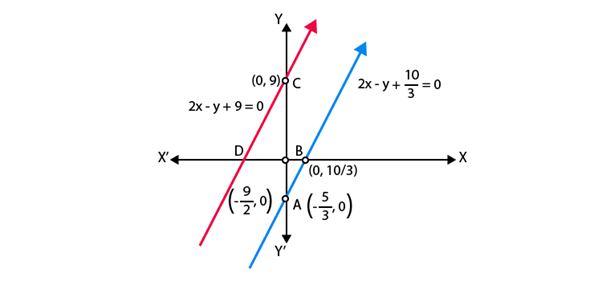Hence, the pair of equations represents two parallel lines.

2. The pair of equations x + 2y + 5 = 0 and –3x – 6y + 1 = 0 have
(a) a unique solution
(b) exactly two solutions
(c) infinitely many solutions
(d) no solution
Ans:
(d)
Explanation:
The equations are:
+ 2+ 5 = 0
–3– 6y + 1 = 0
a= 1; b1 = 2; c= 5
a= -3; b2 = -6; c= 1
a1/a= -1/3
b1/b2 = -2/6 = -1/3
c1/c= 5/1 = 5
Here,
a1/a= b1/b2 ≠ c1/c2
Therefore, the pair of equation has no solution.

3. If a pair of linear equations is consistent, then the lines will be
(a) parallel
(b) always coincident
(c) intersecting or coincident
(d) always intersecting
Ans: (c)
Explanation:
Condition for a pair of linear equations to be consistent are:
Intersecting lines having unique solution,
a1/a≠ b1/b2
Coincident or dependent
a1/a= b1/b2 = c1/c2

4. The pair of equations y = 0 and y = –7 has
(a) one solution
(b) two solutions
(c) infinitely many solutions
(d) no solution

Ans: (d)
Explanation:
The given pair of equations are y = 0 and y = – 7.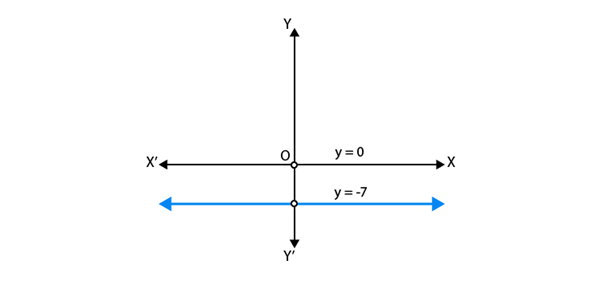Graphically, both lines are parallel and have no solution

5. The pair of equations x = a and y = b graphically represents lines which are
(a) parallel
(b) intersecting at (b, a)
(c) coincident
(d) intersecting at (a, b)
Ans:
(d)
Explanation:
Graphically in every condition,
a, b>>0
a, b< 0
a>0, b< 0
a<0, b>0 but a = b≠ 0.
The pair of equations x = a and y = b graphically represents lines which are intersecting
at (a, b).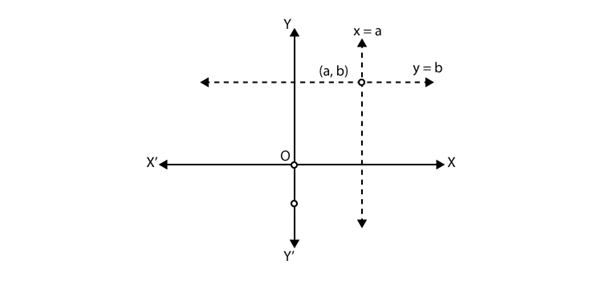Hence, the cases two lines intersect at (a, b).

Exercise 3.2

1. Do the following pair of linear equations have no solution? Justify your answer.
(i) 2x + 4y = 3
12y + 6x = 6
(ii) x = 2y
y = 2x
(iii) 3x + y – 3 = 0
2x + 2/3y = 2
Solution:

The Condition for no solution = a1/a= b1/b2 ≠ c1/c2 (parallel lines)
(i) Yes.
Given pair of equations are,
2x + 4y – 3 = 0 and 6x + 12y – 6 = 0
Comparing the equations with ax+ by +c = 0;
We get,
a1 = 2, b1 = 4, c1 = – 3;
a2 = 6, b2 = 12, c2 = – 6;
a1 /a2 = 2/6 = 1/3
b1 /b2 = 4/12 = 1/3
c1 /c2 = – 3/ – 6 = ½
Here, a1/a2 = b1/b≠ c1/c2, i.e parallel lines
Hence, the given pair of linear equations has no solution.
(ii) No.
Given pair of equations,
x = 2y or x – 2y = 0
y = 2x or 2x – y = 0;
Comparing the equations with ax+ by +c = 0;
We get,
a1 = 1, b1 = – 2, c1 = 0;
a2 = 2, b2 = – 1, c2 = 0;
a1 /a2 = ½
b1 /b2 = -2/-1 = 2
Here, a1/a2 ≠ b1/b2.
Hence, the given pair of linear equations has unique solution.
(iii) No.
Given pair of equations,
3x + y – 3 = 0
2x + 2/3 y = 2
Comparing the equations with ax+ by +c = 0;
We get,
a1 = 3, b1 = 1, c1 = – 3;
a2 = 2, b2 = 2/3, c2 = – 2;
a1 /a2 = 2/6 = 3/2
b1 /b2 = 4/12 = 3/2
c1 /c2 = – 3/-2 = 3/2
Here, a1/a2 = b1/b2 = c1/c2, i.e coincident lines

2. Do the following equations represent a pair of coincident lines? Justify your answer.
(i) 3x + 1/7y = 3
7x + 3y = 7
(ii) –2x – 3y = 1
6y + 4x = – 2
(iii) x/2 + y + 2/5 = 0
4x + 8y + 5/16 = 0
Solution:

Condition for coincident lines,
a1/a2 = b1/b2 = c1/c2;
(i) No.
Given pair of linear equations are:
3x + 1/7y = 3
7x + 3y = 7
Comparing the above equations with ax + by + c = 0;
Here, a1 = 3, b1 = 1/7, c1 = – 3;
And a2 = 7, b2 = 3, c2 = – 7;
a1 /a2 = 3/7
b1 /b2 = 1/21
c1 /c2 = – 3/ – 7 = 3/7
Here, a1/a2 ≠ b1/b2.
Hence, the given pair of linear equations has unique solution.
(ii) Yes,
Given pair of linear equations.
– 2x – 3y – 1 = 0 and 4x + 6y + 2 = 0;
Comparing the above equations with ax + by + c = 0;
Here, a1 = – 2, b1 = – 3, c1 = – 1;
And a2 = 4, b2 = 6, c2 = 2;
a1 /a2 = – 2/4 = – ½
b1 /b2 = – 3/6 = – ½
c1 /c2 = – ½
Here, a1/a2 = b1/b2 = c1/c2, i.e. coincident lines
Hence, the given pair of linear equations is coincident.
(iii) No,
Given pair of linear equations are
x/2 + y + 2/5 = 0
4x + 8y + 5/16 = 0
Comparing the above equations with ax + by + c = 0;
Here, a1 = ½, b1 = 1, c1 = 2/5;
And a2 = 4, b2 = 8, c2 = 5/16;
a1 /a2 = 1/8
b1 /b2 = 1/8
c1 /c2 = 32/25
Here, a1/a2 = b1/b≠ c1/c2, i.e. parallel lines
Hence, the given pair of linear equations has no solution.

3. Are the following pair of linear equations consistent? Justify your answer.
(i) –3x– 4y = 12
4y + 3x = 12
(ii) (3/5)x – y = ½
(1/5)x – 3y= 1/6
(iii) 2ax + by = a
1. ax + 2by – 2a = 0; a, b ≠ 0
(iv) x + 3y = 11
2 (2x + 6y) = 22
Solution:

Conditions for pair of linear equations to be consistent are:
a1/a2 ≠ b1/b2. [unique solution]
a1/a2 = b1/b2 = c1/c2 [coincident or infinitely many solutions]
(i) No.
The given pair of linear equations
– 3x – 4y – 12 = 0 and 4y + 3x – 12 = 0
Comparing the above equations with ax + by + c = 0;
We get,
a1 = – 3, b1 = – 4, c1 = – 12;
a2 = 3, b2 = 4, c2 = – 12;
a1 /a2 = – 3/3 = – 1
b1 /b2 = – 4/4 = – 1
c1 /c2 = – 12/ – 12 = 1
Here, a1/a2 = b1/b≠ c1/c2
Hence, the pair of linear equations has no solution, i.e., inconsistent.
(ii) Yes.
The given pair of linear equations
(3/5)x – y = ½
(1/5)x – 3y= 1/6
Comparing the above equations with ax + by + c = 0;
We get,
a1 = 3/5, b1 = – 1, c1 = – ½;
a2 = 1/5, b2 = 3, c2 = – 1/6;
a1 /a2 = 3
b1 /b2 = – 1/ – 3 = 1/3
c1 /c2 = 3
Here, a1/a2 ≠ b1/b2.
Hence, the given pair of linear equations has unique solution, i.e., consistent.
(iii) Yes.
The given pair of linear equations –
2ax + by –a = 0 and 4ax + 2by – 2a = 0
Comparing the above equations with ax + by + c = 0;
We get,
a1 = 2a, b1 = b, c1 = – a;
a2 = 4a, b2 = 2b, c2 = – 2a;
a1 /a2 = ½
b1 /b2 = ½
c1 /c2 = ½
Here, a1/a2 = b1/b2 = c1/c2
Hence, the given pair of linear equations has infinitely many solutions, i.e., consistent
(iv) No.

The given pair of linear equations
x + 3y = 11 and 2x + 6y = 11
Comparing the above equations with ax + by + c = 0;
We get,
a1 = 1, b1 = 3, c1 = 11
a2 = 2, b2 = 6, c2 = 11
a1 /a2 = ½
b1 /b2 = ½
c1 /c2 = 1
Here, a1/a2 = b1/b≠ c1/c2.
Hence, the given pair of linear equations has no solution.

Exercise 3.3

1. For which value(s) of λ, do the pair of linear equations
λx + y = λ2 and x + λy = 1 have
(i) no solution?
(ii) infinitely many solutions?
(iii) a unique solution?
Solution:

The given pair of linear equations is
λx + y = λ2 and x + λy = 1
a1 = λ, b1= 1, c1 = – λ2
a2 = 1, b= λ, c2= -1
The given equations are;
λ x + y – λ2 = 0
x + λ y – 1 = 0
Comparing the above equations with ax + by + c = 0;
We get,
a1 = λ, b1 = 1, c1 = – λ 2;
a2 = 1, b2 = λ, c2 = – 1;
a1 /a2 = λ/1
b1 /b2 = 1/λ
c1 /c2 = λ2
(i) For no solution,
a1/a2 = b1/b2≠ c1/c2
i.e. λ = 1/ λ ≠ λ2
so, λ 2 = 1;
and λ 2 ≠ λ
Here, we take only λ = – 1,
Since the system of linear equations has no solution.
(ii) For infinitely many solutions,
a1/a2 = b1/b2 = c1/c2
i.e. λ = 1/ λ  = λ2
so λ = 1/ λ gives λ = + 1;
λ = λ 2 gives λ = 1,0;
Hence satisfying both the equations
λ = 1 is the answer.
(iii) For a unique solution,
a1/a2 ≠ b1/b2
so λ ≠1/ λ
hence, λ2 ≠ 1;
λ ≠ + 1;
So, all real values of λ except +1

2. For which value(s) of will the pair of equations
kx + 3= – 3
12+ ky = k
have no solution?
Solution:
The given pair of linear equations is
kx + 3y = k – 3 …(i)
12x + ky = k …(ii)
On comparing the equations (i) and (ii) with ax + by = c = 0,
We get,
a1 = k, b1 = 3, c1 = -(k – 3)
a2 = 12, b2 = k, c2 = – k
Then,
a1 /a2 = k/12
b1 /b2 = 3/k
c1 /c2 = (k-3)/k
For no solution of the pair of linear equations,
a1/a2 = b1/b2≠ c1/c2
k/12 = 3/k ≠ (k-3)/k
Taking first two parts, we get
k/12 = 3/k
k2 = 36
k = + 6
Taking last two parts, we get
3/k ≠ (k-3)/k
3k ≠ k(k – 3)
k2 – 6k ≠ 0
so, k ≠ 0, 6
Therefore, value of k for which the given pair of linear equations has no solution is k = – 6.

3. For which values of and b, will the following pair of linear equations have infinitely many solutions?
+ 2= 1
(b)+ (+ b)= + – 2
Solution:
The given pair of linear equations are:
x + 2y = 1 …(i)
(a - b)x + (a + b)y = a + b – 2 …(ii)
On comparing with ax + by = c = 0 we get
a1 = 1, b1 = 2, c1 = – 1
a2 = (a – b), b2 = (a + b), c2 = – (a + b – 2)
a1 /a2 = 1/(a - b)
b1 /b2 = 2/(a + b)
c1 /c2 = 1/(a + b - 2)
For infinitely many solutions of the, pair of linear equations,
a1/a2 = b1/b2=c1/c2(coincident lines)
so, 1/(a - b) = 2/ (a + b) = 1/(a + b - 2)
Taking first two parts,
1/(a-b) = 2/ (a+b)
a + b = 2(a – b)
a = 3b …(iii)
Taking last two parts,
2/ (a + b) = 1/(a + b - 2)
2(a + b – 2) = (a + b)
a + b = 4 …(iv)
Now, put the value of a from Eq. (iii) in Eq. (iv), we get
3b + b = 4
4b = 4
b = 1
Put the value of b in Eq. (iii), we get
a = 3
So, the values (a, b) = (3, 1) satisfies all the parts. Hence, required values of a and b are 3 and 1 respectively for which the given pair of linear equations has infinitely many solutions.

4. Find the value(s) of p in (i) to (iv) and p and q in (v) for the following pair of equations:
(i) 3x – y – 5 = 0 and 6x – 2y – p = 0, if the lines represented by these equations are parallel.
Solution:
Given pair of linear equations is
3x – y – 5 = 0 …(i)
6x – 2y – p = 0 …(ii)
On comparing with ax + by + c = 0 we get
We get,
a1 = 3, b1 = – 1, c1 = – 5;
a2 = 6, b2 = – 2, c2 = – p;
a1 /a2 = 3/6 = ½
b1 /b2 = ½
c1 /c2 = 5/p
Since, the lines represented by these equations are parallel, then
a1/a2 = b1/b≠ c1/c2
Taking last two parts, we get ½ ≠ 5/p
So, p ≠ 10
Hence, the given pair of linear equations are parallel for all real values of p except 10.

(ii) – + py = 1 and px = 1, if the pair of equations has no solution.
Solution:
Given pair of linear equations is
– x + py = 1 …(i)
px – y – 1 = 0 …(ii)
On comparing with ax + by + c = 0,
We get,
a1 = -1, b1 = p, c1 =- 1;
a2 = p, b2 = – 1, c2 =- 1;
a1 /a2 = -1/p
b1 /b2 = – p
c1 /c2 = 1
Since, the lines equations has no solution i.e., both lines are parallel to each other.
a1/a2 = b1/b2≠ c1/c2
-1/p = – p ≠ 1
Taking last two parts, we get
p ≠ -1
Taking first two parts, we get
p2 = 1
p = + 1
Hence, the given pair of linear equations has no solution for p = 1.

(iii) – 3+ 5= 7 and 2px – 3= 1, if the lines represented by these equations are intersecting at a unique point.
Solution:
Given, pair of linear equations is
– 3x + 5y = 7
2px – 3y = 1
On comparing with ax + by + c = 0, we get
Here, a1 = -3, b1 = 5, c1 = – 7;
And a2 = 2p, b2 = – 3, c2 = – 1;
a1 /a2 = -3/ 2p
b1 /b2 = – 5/3
c1 /c2 = 7
Since, the lines are intersecting at a unique point i.e., it has a unique solution
a1/a2 ≠ b1/b2
-3/2p ≠ -5/3
p ≠ 9/10
Hence, the lines represented by these equations are intersecting at a unique point for all real values of p except 9/10

(iv) 2+ 3– 5 = 0 and px – 6– 8 = 0, if the pair of equations has a unique solution.
Solution:
Given, pair of linear equations is
2x + 3y – 5 = 0
px – 6y – 8 = 0
On comparing with ax + by + c = 0 we get
Here, a1 = 2, b1 = 3, c1 = – 5;
And a2 = p, b2 = – 6, c2 = – 8;
a1 /a2 = 2/p
b1 /b2 = – 3/6 = – ½
c1 /c2 = 5/8
Since, the pair of linear equations has a unique solution.
a1/a2 ≠ b1/b2
so 2/p ≠ – ½
p ≠ – 4
Hence, the pair of linear equations has a unique solution for all values of p except – 4.

(v) 2+ 3= 7 and 2px + py = 28 – qy, if the pair of equations have infinitely many solutions.
Solution:
Given pair of linear equations is
2x + 3y = 7
2px + py = 28 – qy
or 2px + (p + q)y – 28 = 0
On comparing with ax + by + c = 0,
We get,
Here, a1 = 2, b1 = 3, c1 = – 7;
And a2 = 2p, b2 = (p + q), c2 = – 28;
a1/a2 = 2/2p
b1/b= 3/ (p + q)
c1/c= ¼
Since, the pair of equations has infinitely many solutions i.e., both lines are coincident.
a1/a2 = b1/b2 = c1/c2
1/p = 3/(p+q) = ¼
Taking first and third parts, we get
p = 4
Again, taking last two parts, we get
3/(p+q) = ¼
p + q = 12
Since p = 4
So, q = 8
Here, we see that the values of p = 4 and q = 8 satisfies all three parts.
Hence, the pair of equations has infinitely many solutions for all values of p = 4 and q = 8.

5. Two straight paths are represented by the equations – 3= 2 and –2+ 6= 5.Check whether the paths cross each other or not.
Solution:
Given linear equations are
x – 3y – 2 = 0 …(i)
-2x + 6y – 5 = 0 …(ii)
On comparing with ax + by c = 0,
We get
a1 = 1, b1 = -3, c1 = - 2;
a2 = -2, b2 = 6, c2 = - 5;
a1/a2 = – ½
b1/b2 = – 3/6 = – ½
c1/c2 = 2/5
i.e., a1/a2 = b1/b2 ≠ c1/c2 [parallel lines]
Hence, two straight paths represented by the given equations never cross each other, because they are parallel to each other.

6. Write a pair of linear equations which has the unique solution = – 1, =3. How many such pairs can you write?
Solution:
Condition for the pair of system to have unique solution
a1/a2 ≠ b1/b2
Let the equations be,
a1x + b1y + c1 = 0
a2x + b2y + c2 = 0
Since, x = – 1 and y = 3 is the unique solution of these two equations, then
It must satisfy the equations –
a1(-1) + b1(3) + c1 = 0
– a1 + 3b1 + c1 = 0 …(i)
and a2(- 1) + b2(3) + c2 = 0
– a2 + 3b2 + c2 = 0 …(ii)
Since for the different values of a1, b1, c1 and a2, b2, c2 satisfy the Eqs. (i) and (ii).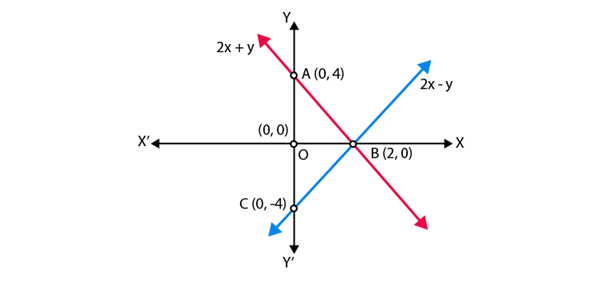Hence, infinitely many pairs of linear equations are possible.

7. If 2+ = 23 and 4= 19, find the values of 5– 2and y/x – 2.
Solution:
Given equations are
2x + y = 23 …(i)
4x – y = 19 …(ii)
On adding both equations, we get
6x = 42
So, x = 7
Put the value of x in Eq. (i), we get
2(7) + y = 23
y = 23 – 14
so, y = 9
Hence 5y – 2x = 5(9) – 2(7) = 45 – 14 = 31
y/x – 2 = 9/7 -2 = -5/7
Hence, the values of (5y – 2x) and y/x – 2 are 31 and -5/7 respectively.

8. Find the values of x and y in the following rectangle [see Fig. 3.2].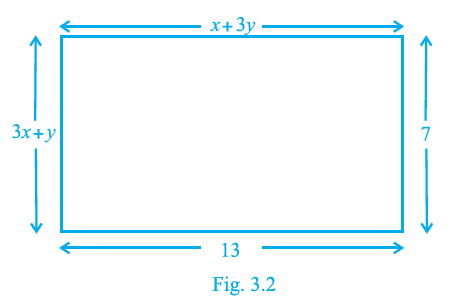Solution:
Using property of rectangle,
We know that,
Lengths are equal,

i.e., CD = AB

Hence, x + 3y = 13 …(i)

Breadth are equal,

i.e., AD = BC

Hence, 3x + y = 7 …(ii)

On multiplying Eq. (ii) by 3 and then subtracting Eq. (i),

We get,

8x = 8

So, x = 1

On substituting x = 1 in Eq. (i),

We get,

y = 4

Therefore, the required values of x and y are 1 and 4, respectively.

Exercise 3.4

1. Graphically, solve the following pair of equations:
2x + y = 6
2x – y + 2 = 0
Find the ratio of the areas of the two triangles formed by the lines representing these equations with the x-axis and the lines with the y-axis.
Solution:

Given equations are 2x + y = 6 and 2x – y + 2 = 0
Table for equation 2x + y – 6 = 0, for x = 0, y = 6, for y = 0, x = 3.

 x 0 3 y 6 0

Table for equation 2x – y + 2 = 0, for x = 0, y = 2, for y = 0,x = – 1

 x 0 -1 y 2 0

Let A1 and A2 represent the areas of triangles ACE and BDE respectively.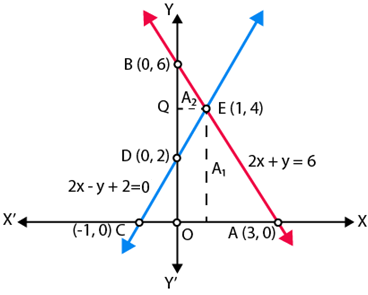Let, Area of triangle formed with x -axis = T1
T1 = Area of △ACE = ½ × AC × PE
T1 = ½ × 4 × 4 = 8
And Area of triangle formed with y – axis = T2
T1 = Area of △BDE = ½ × BD × QE
T1 = ½ × 4 × 1 = 2
T1:T2 = 8:2 = 4:1
Hence, the pair of equations intersect graphically at point E(1, 4)
i.e., x = 1 and y = 4.

2. Determine, graphically, the vertices of the triangle formed by the lines
y = x, 3y = x, x + y = 8
Solution:
Given linear equations are
y = x …(i)
3y = x …(ii)
and x + y = 8 …(iii)
Table for line y = x,

 x 0 1 2 y 0 1 2

Table for line x = 3y,

 x 0 3 6 y 0 1 2

Table for line x + y = 8

 x 0 4 8 y 8 4 0

Plotting the points A (1, 1), B(2, 2), C (3, 1), D (6, 2), we get the straight lines AB and CD.
Similarly, plotting the point P (0, 8), Q(4, 4) and R(8, 0), we get the straight line PQR.
AB and CD intersects the line PR on Q and D, respectively.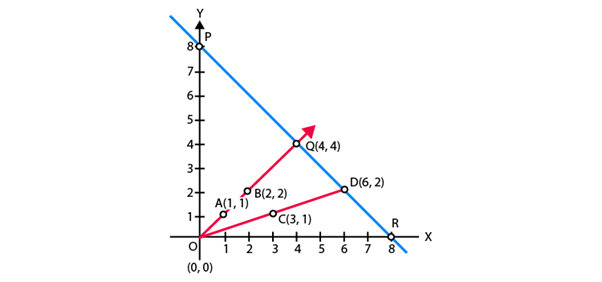So, △OQD is formed by these lines. Hence, the vertices of the △OQD formed by the given lines are O(0, 0), Q(4, 4) and D(6, 2).

3. Draw the graphs of the equations x = 3, x = 5 and 2x – y – 4 = 0. Also find the area of the quadrilateral formed by the lines and the x–axis.
Solution:
Given equation of lines x = 3, x = 5 and 2x - y - 4 = 0.
Table for line 2x – y – 4 = 0

 x 0 2 y -4 0

Plotting the graph, we get,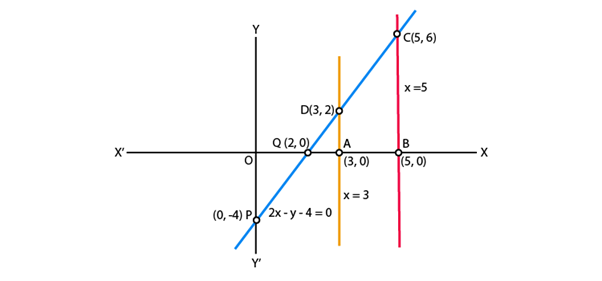From the graph, we get,
AB = OB-OA = 5-3 = 2
AD = 2
BC = 6
Thus, quadrilateral ABCD is a trapezium, then,
Area of Quadrileral ABCD = ½ × (distance between parallel lines) = ½ × (AB) × (AD + BC)
= 8 sq units

4. The cost of 4 pens and 4 pencil boxes is Rs 100. Three times the cost of a pen is Rs 15 more than the cost of a pencil box. Form the pair of linear equations for the above situation. Find the cost of a pen and a pencil box.
Solution:
Let the cost of a pen and a pencil box be Rs x and Rs y respectively.
According to the question,
4x + 4y = 100
Or x + y = 25 …(i)
3x = y + 15
Or 3x - y = 15 …(ii)
On adding Equation (i) and (ii), we get,
4x = 40
So, x = 10
Substituting x = 10, in Eq. (i) we get
y = 25 - 10 = 15
Hence, the cost of a pen = Rs. 10
The cost of a pencil box = Rs. 15

5. Determine, algebraically, the vertices of the triangle formed by the lines
3= 3
2– 3= 2
+ 2= 8
Solution:
3x – y = 2 …(i)
2x -3y = 2 …(ii)
x + 2y = 8 …(iii)
Let the equations of the line (i), (ii) and (iii) represent the side of a ∆ABC.
On solving (i) and (ii),
We get,
[First, multiply Eq. (i) by 3 in Eq. (i) and then subtract]
(9x - 3y)-(2x - 3y) = 9 - 2
7x = 7
x = 1
Substituting x=1 in Eq. (i), we get
3 × 1 - y = 3
y = 0
So, the coordinate of point B is (1, 0)
On solving lines (ii) and (iii),
We get,
[First, multiply Eq. (iii) by 2 and then subtract]
(2x + 4y)-(2x-3y) = 16-2
7y = 14
y = 2
Substituting y=2 in Eq. (iii), we get
x + 2 × 2 = 8
x + 4 = 8
x = 4
Hence, the coordinate of point C is (4, 2).
On solving lines (iii) and (i),
We get,
[First, multiply in Eq. (i) by 2 and then add]
(6x - 2y) + (x + 2y) = 6 + 8
7x = 14
x = 2
Substituting x=2 in Eq. (i), we get
3 × 2 – y = 3
y = 3
So, the coordinate of point A is (2, 3).
Hence, the vertices of the ∆ABC formed by the given lines are as follows,
A (2, 3), B (1, 0) and C (4, 2).

6. Ankita travels 14 km to her home partly by rickshaw and partly by bus. She takes half an hour if she travels 2 km by rickshaw, and the remaining distance by bus.
Solution:
Let the speed of the rickshaw and the bus are x and y km/h, respectively.
Now, she has taken time to travel 2 km by rickshaw, t1 = (2/x) hr
Speed = distance/ time
she has taken time to travel remaining distance i.e., (14 – 2) = 12km
By bus t2 = (12/y) hr
By first condition,
t+ t2 = ½ = (2/x) + (12/y) … (i)
Now, she has taken time to travel 4 km by rickshaw, t3 = (4/x) hr
and she has taken time to travel remaining distance i.e., (14 – 4) = 10km, by bus = t4 = (10/y) hr
By second condition,
t3 + t4 = ½ + 9/60 = ½ + 3/20
(4/x) + (10/y) = (13/20) …(ii)
Let (1/x) = u and (1/y) = v
Then Equations. (i) and (ii) becomes
2u + 12v = ½ …(iii)
4u + 10v = 13/20…(iv)
[First, multiply Eq. (iii) by 2 and then subtract]
(4u + 24v) – (4u + 10v) = 1–13/20
14v = 7/20
v = 1/40
Substituting the value of v in Eq. (iii),
2u + 12(1/40) = ½
2u = 2/10
u = 1/10
x = 1/u = 10km/hr
y = 1/v = 40km/hr
Hence, the speed of rickshaw = 10 km/h
And the speed of bus = 40 km/h.

Offer running on EduRev: Apply code STAYHOME200 to get INR 200 off on our premium plan EduRev Infinity!

## Mathematics (Maths) Class 10

51 videos|346 docs|103 tests

,

,

,

,

,

,

,

,

,

,

,

,

,

,

,

,

,

,

,

,

,

;